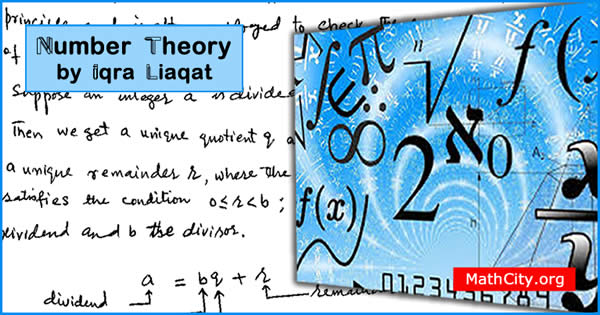# Number Theory by Ms. Iqra LiaqatNotes of number theory provided Ms. Iqra Liaqat is a very good addition in the MSc notes section. We are actually quite grateful to her for giving these notes and likes her encouragement to distribute these notes on MathCity.org

 Name Number Theory Ms. Iqra Liaqat 280 pages PDF (see Software section for PDF Reader) 3.06 mB

In this section, the main heading are given below.

• Well ordering principle (WOP)
• Archimedian property
• Multiple
• Divisibility
• The floor function and ceiling function
• The division algorithm
• Div and mod operators
• Basic representation theorem
• Greatest common divisor
• Euclid's theorem
• Sieve of eratosthenes
• Twin primes
• Mersenn number
• Division algorithm
• Euclid's lemma
• Lease common multiple
• Greated common divisor
• Relation between GCD and LCM
• Linear Diophantine equation
• Congruences
• Linear congruence
• Continuous fraction
• Polynomial congruence
• Factor theorem
• Lagrange's theorem
• Euler's theorem
• Wilson's theorem

• msc/notes/number-theory-iqra-liaqat